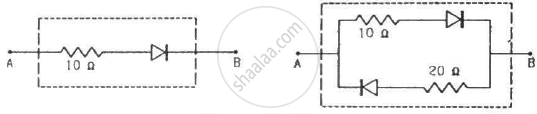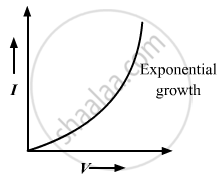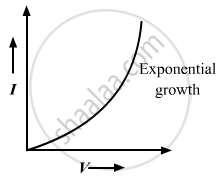Advertisement Remove all ads

# Draw the Current-voltage Characteristics for the Device Show in Figure Between the Terminals a and B. - Physics

Short Note

Draw the current-voltage characteristics for the device show in figure between the terminals A and B.(Assume that the resistance of each diode is zero in forward bias and is infinity in reverse bias.)

Advertisement Remove all ads

#### Solution

(a) If a battery is connected between terminals A and B, with positive terminal connected to point A and negative terminal connected to point B, then the diode will get forward biassed by the applied voltage. So, the current voltage graph for this circuit will be the same as that of the characteristic curves of a forward-biassed diode.(b) If a battery is connected between terminals A and B, with positive terminal connected to point A and negative terminal connected to point B, then the upper diode will get forward biassed and the lower diode will get reverse biassed by the applied voltage. So, this lower branch can be replaced by an open circuit; hence, the current flow through this branch will be zero. The current flows only through the upper diode, so the circuit on simplification will become identical to the circuit in part (a). Hence, the current voltage graph for this circuit will be the same as that of the characteristic curves of a forward-biassed diode.Concept: p-n Junction
Is there an error in this question or solution?
Advertisement Remove all ads

#### APPEARS IN

HC Verma Class 11, Class 12 Concepts of Physics Vol. 2
Chapter 23 Semiconductors and Semiconductor Devices
Q 29 | Page 420
Advertisement Remove all ads
Advertisement Remove all ads
Share
Notifications

View all notifications

Forgot password?